# Difference between revisions of "Uniformly bounded derivatives implies globally analytic"

## Statement

### Global statement

Suppose$f$ is an infinitely differentiable function on$\R$ such that, for any fixed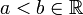$a, there is a constant$C$ (possibly dependent on$a,b$) such that for all nonnegative integers$n$, we have: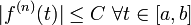$|f^{(n)}(t)| \le C \ \forall t \in [a,b]$

Then,$f$ is a globally analytic function: the Taylor series of$f$ about any point in$\R$ converges to$f$. In particular, the Taylor series of$f$ about 0 converges to$f$.

## Facts used

1. Max-estimate version of Lagrange formula

## Examples

The functions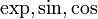$\exp, \sin, \cos$ all fit this description.

If$f = \exp$, we know that each of the derivatives equals$\exp$, so$f^{(n)}(t) = f(t)$ for all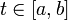$t \in [a,b]$. Since$\exp$ is continuous, it is bounded on the closed interval$[a,b]$, and the upper bound for$\exp$ thus serves as a uniform bound for all its derivatives. (In fact, since$f$ is increasing, we can explicitly take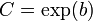$C = \exp(b)$).

For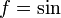$f = \sin$ or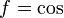$f = \cos$, we know that all the derivatives are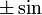$\pm \sin$ or$\pm \cos$, so their magnitude is at most 1. Thus, we can take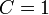$C = 1$.

## Proof

Given:$f$ is an infinitely differentiable function on$\R$ such that, for any fixed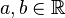$a,b \in \R$, there is a constant$C$ (possibly dependent on$a,b$) such that for all nonnegative integers$n$, we have:$|f^{(n)}(t)| \le C \ \forall t \in [a,b]$

A point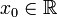$x_0 \in \R$ and a point$x \in \R$.

To prove: The Taylor series of$f$ at$x_0$, evaluated at$x$, converges to$f(x)$.

Proof: Note that if$x_0 = x$, there is nothing to prove, so we consider the case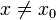$x \ne x_0$.

In order to show this, it suffices to show that$\lim_{n \to \infty} P_n(f;x_0)(x) = f(x)$ where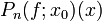$P_n(f;x_0)(x)$ denotes the$n^{th}$ Taylor polynomial of$f$ at$x_0$, evaluated at$x$.

This in turn is equivalent to showing that the remainder approaches zero:

'Want to show: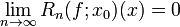$\lim_{n \to \infty} R_n(f;x_0)(x) = 0$

where$R_n(f;x_0)(x) = f(x) - P_n(f;x_0)(x)$.

Proof of what we want to show: By Fact (1), we have that:$|R_n(f;x_0)(x)| \le \left(\max_{t \in J} |f^{(n+1)}(t)|\right) \frac{|x - x_0|^{n+1}}{(n + 1)!}$

where$J$ is the interval joining$x_0$ to$x$. Let$a = \min \{ x,x_0 \}$ and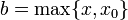$b = \max \{ x, x_0 \}$. The interval$J$ is the interval$[a,b]$.

Now, from the given data, there exists$C$, dependent on$x$ and$x_0$ but not on$n$, such that:$\max_{t \in J} |f^{(n+1)}(t)| \le C \ \forall \ n$

Plugging this in, we get that:$|R_n(f;x_0)(x)| \le C \frac{|x - x_0|^{n+1}}{(n + 1)!}$

Now taking the limit as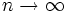$n \to \infty$, we get:$\lim_{n \to \infty} |R_n(f;x_0)(x)| \le C \lim_{n \to \infty} \frac{|x - x_0|^{n+1}}{(n + 1)!}$

Since exponentials grow faster than power functions, the expression under the limit goes to zero, so we are left with a right side of zero, hence the left side limit is zero, and we are done.